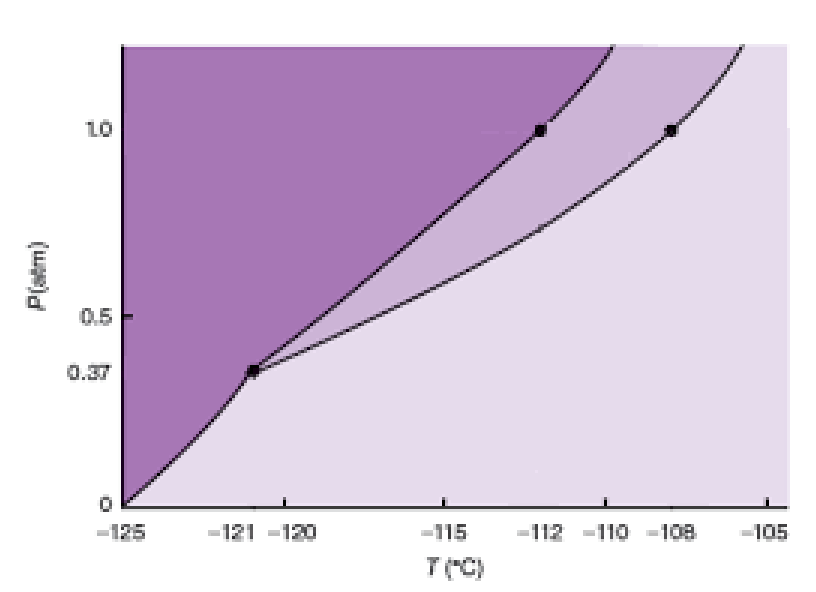Chemistry Practice Problems Phase Diagram Practice Problems Solution: The phase diagram for xenon is shown below:Which i...

# Solution: The phase diagram for xenon is shown below:Which is the denser phase; solid or liquid? Explain your answer.

###### Problem

The phase diagram for xenon is shown below:###### Solution

Analyze the given phase diagram and destablish the relationship of density and the boundary for solid-liquid phase in phase diagram

Recall that a phase diagram shows the transition of matter between solid, liquid, and gas phases as temperature and pressure changes.

In a phase diagram, the different phases can be identified by their location:

• Solid: can be found at high pressure and low temperature

• Liquid: can be found between the solid and gas regions

• Gas: can be found at low pressure and high temperatureView Complete Written Solution

Phase Diagram

Phase Diagram

#### Q. Which of the following statements is true?A. The triple point of a substance increases when the pressure increases.B. The critical point of a substanc...

Solved • Fri Dec 20 2019 08:29:26 GMT-0500 (EST)

Phase Diagram

#### Q. Starting at Point A on the Phase Diagram (where CO2 coexists as a solid and liquid), if the pressure is steadily decreased all the way to 1.0 atm, but...

Solved • Tue Nov 26 2019 12:15:57 GMT-0500 (EST)

Phase Diagram

#### Q. Which point on the phase diagram represents the triple point of CO2?A) AB) BC) CD) DE) X

Solved • Tue Nov 26 2019 12:08:44 GMT-0500 (EST)

Phase Diagram

#### Q. During which of the following phase changes must heat be added to overcome intermolecular forces?I. VaporizationII. SublimationIII. DepositionA) I onl...

Solved • Tue Nov 26 2019 11:38:14 GMT-0500 (EST)